Courses

# Test: General Organic Chemistry Level - 2

## 30 Questions MCQ Test Organic Chemistry | Test: General Organic Chemistry Level - 2

Description
This mock test of Test: General Organic Chemistry Level - 2 for Chemistry helps you for every Chemistry entrance exam. This contains 30 Multiple Choice Questions for Chemistry Test: General Organic Chemistry Level - 2 (mcq) to study with solutions a complete question bank. The solved questions answers in this Test: General Organic Chemistry Level - 2 quiz give you a good mix of easy questions and tough questions. Chemistry students definitely take this Test: General Organic Chemistry Level - 2 exercise for a better result in the exam. You can find other Test: General Organic Chemistry Level - 2 extra questions, long questions & short questions for Chemistry on EduRev as well by searching above.
QUESTION: 1

Solution:
QUESTION: 2

Solution:
QUESTION: 3

### Which of the following is most basic?

Solution:
QUESTION: 4

The total number of cyclic isomers possible for a hydrocarbon with the molecular formula C4H6 is

Solution:

5 cyclic isomers are possible for C4H6, possible.

QUESTION: 5

The correct order of acidity of the following compound A-C is: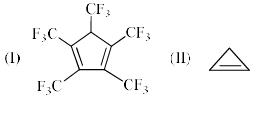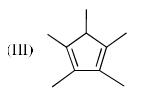Solution:

It follows huckels rule. in the 1st case if it loses H+ ion then it's conjugate base is stabilized by all the CF3 groups . in 3rd case conjugate base is stabilized by electron withdrawing double bonds.hence stable. in 2nd case it becomes antiaromatic.

QUESTION: 6

The order of acidity of the protons H in each of the following is: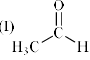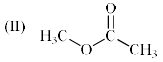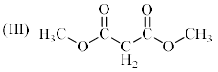Solution:

Aldehyde is more acidic then ester, as ther is +R effect of -OMe group in ester, it decreases the electron withdrawing power of carbonyl group.for second case it is ester.but for 3rd case, the (alfa H) is attached with two carbonyl group.
so the order of acidity is ¡>¡¡¡>¡¡

QUESTION: 7

Imidazole has a pKa = 7 with respect to its conjugate acid. Which N is protonated in this conjugate acid and why?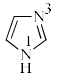Solution:
QUESTION: 8

When CH3Cl undergoes homolytic bond-fission:

Solution:

Explanation :

CH3Cl --------------> CH3 + Cl

Methyl free radical sp2 hybridized (with singly occupied p-orbital.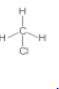(sp3 hybridized carbon) tetrahedral bond angle 109o28

QUESTION: 9

For the following compounds, which nitrogen has the least tendency to be protonated?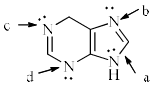Solution: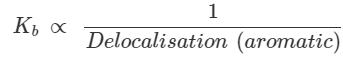QUESTION: 10

Increasing order of pKa values (pKa = –log Ka) of H2O, CH3OH and C6H5OH is:

Solution:

The pKa of water is 14

The pKa of methanol (CH3OH) is 15.5

The pKa of Phenol (C6H5OH) is 9.88

QUESTION: 11

The main sources of arenes among these are:

Solution:

As these petroleum and natural gas comprise of naturally occurring compounds such as carbon and hydrogen in abundance, these serve as the main source of arenes.

QUESTION: 12

Which of the following reaction undergoes in the forward direction:

Solution:

Negative charge over S atom gets additional stability by conjugation through vacent 3d orbital of S ,so HS- is the most stable species and the reaction undergoes in the forward direction.

QUESTION: 13

Which among these is the simplest example for polycyclic arenes?

Solution:

Naphthalene has fused ring of aromaticity and has the simplest structure when compared with other polycyclic aromatic hydrocarbons.

QUESTION: 14

The compound that is anti-aromatic is:

Solution:
QUESTION: 15

The compound A is: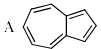Solution:
QUESTION: 16

Which among these is not a representative arene compound?

Solution:

Picric acid is a representative arene compound but not picric chloride.

QUESTION: 17

Identify the correct statement which is related to aromatic hydrocarbon

Solution:

An aromatic hydrocarbon always has a sigma as well as a delocalized pi bond found between the carbon atoms.

QUESTION: 18

Arrange the following compounds in order of decreasing acidity: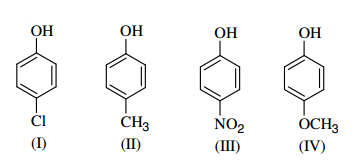Solution:

Electron donating groups increase the acidity of phenol when present as substituent on the benzene ring while electron withdrawing groups decrease the acidity of phenol.

Cl and NO2 are electron withdrawing groups while -CH3 and -OCH3 are electron donating groups.

However, nitro undergoes resonance also which allows it to better stabilize the negative charge of the oxygen as compare to chlorine and thus acidic character of nitro phenol would be higher than that of chloro phenol.

So, Nitrophenol is the most acidic compound here followed by chloro phenol.

Hence, the correct option is 2.

QUESTION: 19

Arrange the following carbocation in increasing order of their stability: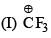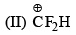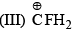Solution:

F shows -I effect and hence it will decrease the stability of carbocation.
so it should be 3>2>1

QUESTION: 20

How many compounds become aromatic after deprotonation?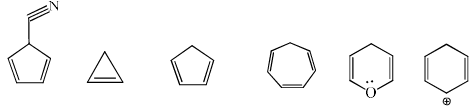Solution:

Compound which gives an stable conjugate base deprotonation will deprotonate easily.

so, 1,3 and 6 will become aromatic after deprotonation.

QUESTION: 21

Which of the following is anti-aromatic?

Solution:
QUESTION: 22

A compound given below shows a large dipole moment. Which of the following resonance structures can be used to adequately explain this observation?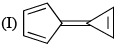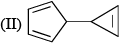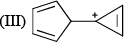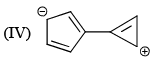Solution:

Both aromatic rings are preferred. Therefore, D option is correct.

QUESTION: 23

Among the following compounds, which is the correct order of % enol content?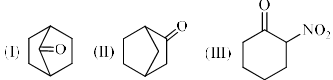Solution:

In the 1st compound the double bond is at the bridgehead carbon , as a result this compound cannot exist in enol form because such conformation would give an impossibility strained ring .Such dis stabilizing effect is absent in 2 nd compound . Whereas the 3 rd compound exclusively exist in enol form due to the formation of intramolecular hydrogen bonding

According to britz's rule at bridge head position -ve charge is unstable so; 3>2>1

QUESTION: 24

Which of the following compounds have higher enol content?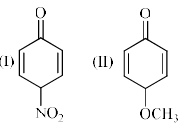Solution:

With electron withdrawing group, the keto-form dominates and with electron donating group, the enol form dominates. Since OCH3 is an electron donating group, (II) will have higher enol content.

QUESTION: 25

Which of the following compounds have less enol content?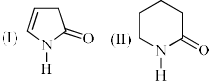Solution:

In the first compound after formation of enol the ring becomes aromatic (when the lonepair over N atom involve in conjugation) and this is a stabilizing factor but no such stabilizing factor is present in the enol form of 2. So 1 has higher enol content and 2 has lower enol content.

QUESTION: 26

The order of number of enolizable protons in each of the following: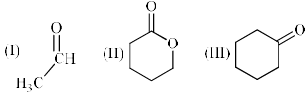Solution:
QUESTION: 27

The amount of enol form present at equilibrium for each of the following is: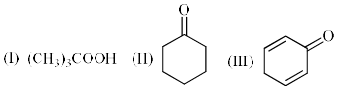Solution:
*Multiple options can be correct
QUESTION: 28

Which is incorrect representation?

Solution:
QUESTION: 29

Arrange the following compounds in acidic order: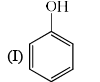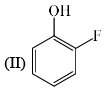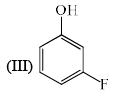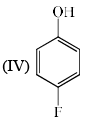Solution:

Halogen group have no mesomeric effect only inductive effect on benzene ring so correct answer is 2>3>4>1

QUESTION: 30

Arrange the following compounds in order of Ca—Cb bond length: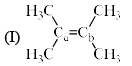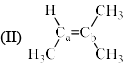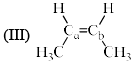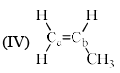Solution: